### A solution to a maximal independent set problem

A distributed system is a set of autonomous computer that comunicate in a network in order to reach a certain goal. So a maximal independent set (MIS) is a distributed system's subject. But, what we intend for MIS?
In graph theory, a maximal independent set or maximal stable set is an independent set that is not a subset of any other independent set.
Some example of MIS are in the graph of cube: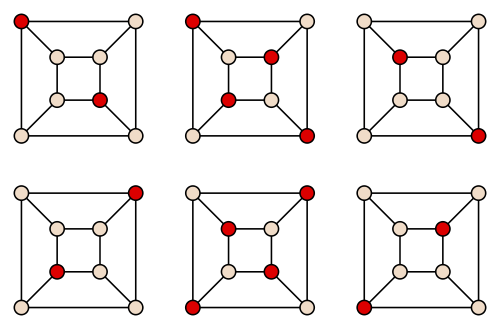(Commons in public domain)
You can see that every maximal independent set is constituted by point that aren't adjacent.
The goal of maximum independet set problem is find the maximum size of the maximal independent set in a given graph or network. In other words the problem is the search of the leaders in a local network of connected processors, and forleaderwe intend an active node connected with an inactive node. This problem is a NP-problem.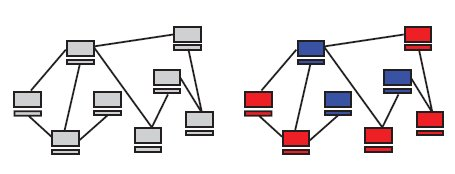Following Afek, Alon, Barad, Hornstein, Barkai and Bar-Joseph,
no methods has been able to efficiently reduce message complexity without assuming knowledge fo the number of neighbours.
But a similar network occurs in the precursors of the fly's sensory bristles, so researchers idea is to use data from this biological network to solve the starting computational problem!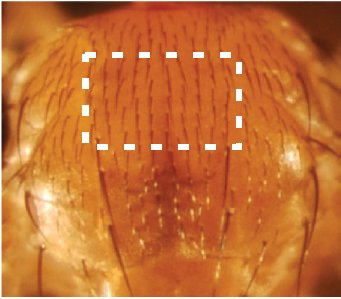Such system is called sensory organ precursors, SOP.
There are a lot of similarities between MIS and SOP:
1. the selection of a particular cell as a SOP is a random event governed by an underlying stochastic process(3, 5);
2. similar to computational requirements SOP selection is probably constrained in time because the default of all cluster cells is to become SOPs unless they are inhibited(4);
3. in computational algorithms(1, 2) processors send messages only when they propose their candidacy to become leaders, thus reducing communication complexity.
In particular this properties are developed in biological network studied using Delta and Notch proteins: in this way a cell selected as SOP inhibits his neighbors obtaining a situation similar to the first figure: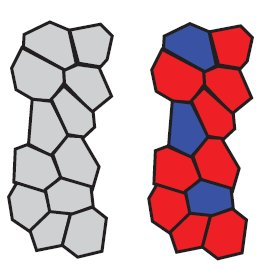Researchers so try to describe the biological network:
We assumed a collection of identical processors placed at nodes of an arbitrary synchronous communication network. Nodes can only broadcast one-bit messages. A message broadcasted by a node reaches all of its neighbors that are still active in the algorithm. In each round, a processor can only tell whether or not a message was sent to it. When a processor receives a message, it cannot tell which of its neighboring processors sent it, and it cannot count the number of messages received in a round.
That in terms of algorithms became: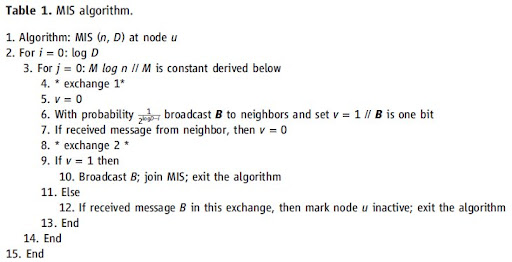where $n$ is the number of nodes, $D$ an upper bound on the number of neighbors any node can have, $M$ a parameter setted to 34, and every node has a probability $p_i$ tosend a message to his neighbors.
In order to select the model Yehuda Afek and collegues confrount experimental data collected from 10 pupas:
between simulated results: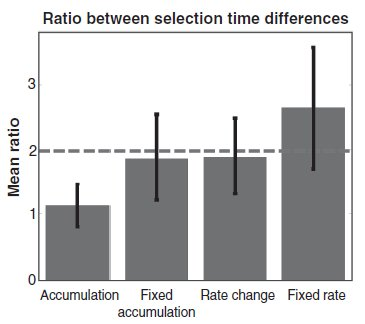And at the end they can conclude that:
the only way the algorithm may err is by terminating while leaving some nodes that are not in A and are also not connected to nodes in A. Next, we show that when the algorithm terminates all nodes are, with high probability, either in A or connected to a node in A, which solves the MIS problem.

(1) M. Luby, SIAM J. Comput. 15, 1036 (1986)
(2) N. Alon, L. Babai, A. Itai, J. Algorithms 7, 567 (1986)
(3) P. Simpson, Curr. Opin. Genet. Dev. 7, 537 (1997)
(4) B. Castro et al., Development 132, 3333 (2005)
(5) M. E. Fortini, Dev. Cell. 16, 633 (2009)

Afek, Y., Alon, N., Barad, O., Hornstein, E., Barkai, N., &amp;amp;amp; Bar-Joseph, Z. (2011). A Biological Solution to a Fundamental Distributed Computing Problem Science, 331 (6014), 183-185 DOI: 10.1126/science.1193210